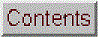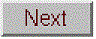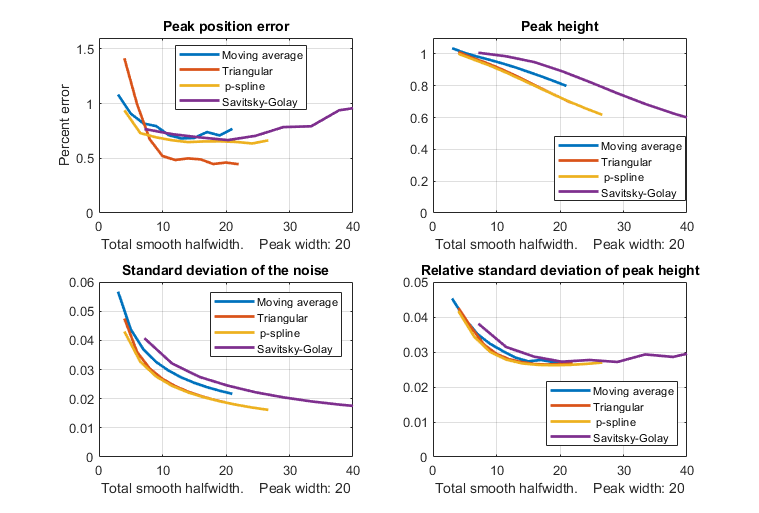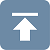[Introduction]  [Signal arithmetic]  [Signals and noise]   [Smoothing]   [Differentiation]  [Peak Sharpening]  [Harmonic analysis]   [Fourier convolution]  [Fourier deconvolution]  [Fourier filter]  [Wavelets]   [Peak area measurement]  [Linear Least Squares]  [Multicomponent Spectroscopy]  [Iterative Curve Fitting]  [Hyperlinear quantitative absorption spectrophotometry] [Appendix and Case Studies]  [Peak Finding and Measurement]  [iPeak]   [iSignal]  [Peak Fitters]   [iFilter]  [iPower]  [List of downloadable software]  [Interactive tools]Smoothing performance comparison

The Matlab/Octave function "MultiPeakOptimization.m" is a self-contained function that compares the performance of four types of linear smooth operations: (1) sliding-average rectangular, (2) triangular, (3) p-spline (equivalent to three passes of a sliding-average), and (4) Savitzky-Golay. These are the four smooth types discussed above, corresponding to the four values of the "SmoothMode" input argument of the ProcessSignal and the interactive iSignal functions. These four smooth operations are applied to a 18000-point signal consisting of 181 Gaussian peaks with a height of 1.0 and a FWHM (full-width at half-maximum) of 20 points ("wid"), line 10), which are all separated by an x-value of 160.01 (line 16), plus added noise consisting of normally-distributed random white noise with a mean of zero and a standard deviation of "Noise" (line 20). The x-axis peak position and y-axis height of each smoothed peak is measured as the height and position of the maximum single signal point for each peak. The relative standard deviation of the measured peak heights is recorded as a function of "total smooth width", tsw, which is the halfwidth of the impulse response of each smooth type (shown in figure 4). The results are shown in the figure below for a peak halfwidth of 20 and a noise standard deviation of 0.2 (i.e., 20% of the peak height).The four quadrants of the graph are: (upper left) peak position error expressed as a percentage of the peak separation; (upper right), the mean peak height of the smoothed peaks; (lower left), the standard deviation of the smoothed noise; and (lower right) the relative standard deviation of the measured peak heights. The different smooth types are indicated by color: blue - sliding-average; red - triangular; yellow - p-spline, and purple - Savitzky-Golay.

These results show that the results of these different smooth types are quite similar but that, the Savitzky-Golay smooth gives the smallest reduction in peak height but the smallest reduction in noise amplitude, compared to the other methods. All these smoothing methods result in similar improvements in the standard deviation of the peak height (bottom right panel) and in the peak position error (upper left panel). Moreover, in all cases, the optimum performance is achieved when the total smooth width is approximately equal to the halfwidth of the peak. The conclusions are the same for a Lorentzian peak, as demonstrated by a similar function "MultiPeakOptimizationLorentzian.m", graphic, the difference being that the peak height reduction is greater for the Lorentzian. For applications where the shape of the signal must be preserved as much as possible, the Savitzky-Golay is the method of choice. In peak detection applications (page 221), on the other hand, where the purpose of smoothing is to reduce the noise in the derivative signal, the retention of the shape of that derivative is less important because peak parameters are determined by least-squares fitting. Therefore, the triangular or p-spline smooth is well suited to this purpose and can be faster for very large smooth widths.

The differences between these methods is even less when the abcissa in the above graph is changed from total smooth bandwidth to noise reduction factor, defined as the square root of the reciprocal of the sum of the square of the impulse response function, as shown in figure window 3.

A more sophisticated method of noise reduction, called wavelet denoising, will be introduced on page 120.Last updated January, 2021. This page is part of "A Pragmatic Introduction to Signal Processing", created and maintained by Prof. Tom O'Haver , Department of Chemistry and Biochemistry, The University of Maryland at College Park. Comments, suggestions and questions should be directed to Prof. O'Haver at toh@umd.edu.
Unique visits since May 17, 2008: